The data in the Diabetes.jmp sample data table consist of measurements on 442 diabetics. The response of interest is Y, disease progression measured one year after a baseline measure was taken. Ten variables thought to be related to disease progression are also measured at baseline. This example shows how to develop a predictive model using generalized regression techniques.
 1 Select Help > Sample Data Library and open Diabetes.jmp.
 2 Select Analyze > Fit Model.
 3 Select Y from the Select Columns list and click Y.
 4 Select Age through Glucose and click Macros > Factorial to degree.
 5 Select Validation from the Select Columns list and click Validation.
 6 From the Personality list, select Generalized Regression.
 7 Click Run.
 8 Click Go.
The Solution Path report (Solution Path Plot) shows plots of the parameter estimates and scaled negative log likelihood. The shrinkage increases as the Magnitude of Scaled Parameter Estimates decreases. The estimates at the far right of the plot are the maximum likelihood estimates. A vertical red line indicates those parameter values selected by the validation criterion, in this case, the holdback sample defined by the column Validation.
Solution Path Plot
 9 Select the option Select Nonzero Terms from the Adaptive Lasso with Validation Column Validation report’s red triangle menu.
This option highlights the nonzero terms in the Parameter Estimates for Original Data report (Portion of Parameter Estimates for Original Predictors Report) and their paths in the Solution Path plot. The corresponding columns in the data table are also selected. Note that only 6 of the 55 parameter estimates are nonzero. Also note that the scale parameter for the normal distribution (sigma) is estimated and shown in the last line of the Parameter Estimates for Original Data report.
Portion of Parameter Estimates for Original Predictors Report
To save the prediction formula, select Save Columns > Save Prediction Formula from the red triangle menu for the Adaptive Lasso with Validation Column Validation report.
Launch the Generalized Regression personality by selecting Analyze > Fit Model, entering one or more columns for Y, and selecting Generalized Regression from the Personality menu (Fit Model Launch Window with Generalized Regression Selected).
Fit Model Launch Window with Generalized Regression Selected
When you select Generalized Regression from the Personality menu, the Distribution option appears. Here you can specify a distribution for Y. The abbreviation ZI means zero-inflated. The distributions are separated into three categories based on their response: continuous, discrete, and zero-inflated. The options are described below.
Y has a normal distribution with mean μ and standard deviation σ. The normal distribution is symmetric and with a large enough sample size, can approximate a large variety of other distributions using the Central Limit Theorem. The link function for μ is the identity. That is, the mean of Y is expressed as a linear model. In estimating the model parameters, the scale parameter σ is profiled out. It is then replaced by its maximum likelihood estimate (MLE). (Note that the MLE is not the typical unbiased estimator for σ.) See Statistical Details for Distributions.
Y has a Cauchy distribution with location parameter μ and scale parameter σ. The Cauchy distribution has an undefined mean and standard deviation. The median and mode are both μ. Most data do not inherently follow a Cauchy distribution, but it is useful for conducting a robust regression on data that contain a large proportion of outliers (up to 50%). The link function for μ and σ is the identity. See Statistical Details for Distributions.
Y has an exponential distribution with mean parameter μ. The exponential distribution is right-skewed and is often used to model lifetimes or the time between successive events. The link function for μ is the logarithm. See Statistical Details for Distributions.
Y has a gamma distribution with mean parameter μ and dispersion parameter σ. The gamma is a flexible distribution and contains a family of other widely used distributions. For example, the exponential distribution is a special case of the gamma distribution where σ = μ. The Weibull and chi squared distributions can also be derived from the gamma distribution. The link function for μ is the logarithm. See Statistical Details for Distributions.
Y has a beta distribution with mean parameter μ and dispersion parameter σ. The response for the beta is between 0 and 1 (not inclusive) and is often used to model proportions or rates. The link function for μ is the logit. See Statistical Details for Distributions.
Quantile Regression
Y has a binomial distribution with parameters p and n. The response, Y, indicates the total number of successes in n independent trials with a fixed probability, p, for all trials. This distribution allows for the use of a sample size column. If no column is listed, it is assumed that the sample size is one. The link function for p is the logit. When you select Binomial as the Distribution, the response variable must be specified in one of the following ways. See Statistical Details for Distributions.
 ‒ Unsummarized: If your data are not summarized as frequencies of events, specify a single binary column as the response.
 ‒
Y has a beta binomial distribution with the probability of success, p, the number of trails, n, and overdispersion parameter, δ. This distribution is an overdispersed version of the binomial distribution.
Run demoBetaBinomial.jsl in the JMP Samples/Scripts folder to compare a beta binomial distribution with dispersion parameter δ to a binomial distribution with parameters p and n = 20.
Y has a Poisson distribution with mean λ. The Poisson distribution typically models the number of events in a given interval and is often expressed as count data. The link function for λ is the logarithm. Poisson regression is permitted even if Y assumes non-integer values. See Statistical Details for Distributions.
Y has a negative binomial distribution with mean μ and dispersion parameter σ. The negative binomial distribution typically models the number of successes before a specified number of failures. The negative binomial distribution is also equivalent to the Gamma Poisson distribution under certain conditions. For more details about the connection between negative binomial and Gamma Poisson, see the Distributions chapter in the Basic Analysis book.
Run demoGammaPoisson.jsl in the JMP Samples/Scripts folder to compare a Gamma Poisson distribution with mean λ and dispersion parameter σ to a Poisson distribution with mean λ.
The link function for μ is the logarithm. Negative binomial regression is permitted even if Y assumes non-integer values. See Statistical Details for Distributions.
Y has a zero-inflated binomial distribution with parameters p, n, and zero-inflation parameter π. The response, Y, indicates the total number of successes in n independent trials with a fixed probability, p, for all trials. This distribution allows for the use of a sample size column. If no column is listed, it is assumed that the sample size is one. The link function for p is the logit. See Statistical Details for Distributions.
Y has a beta binomial distribution with the probability of success, p, the number of trails, n, overdispersion parameter, δ, and zero-inflation parameter π. This distribution is an overdispersed version of the ZI binomial distribution. The ZI beta binomial distribution requires a sample size greater than one for each observation. Thus, the user must specify a sample size column. To insert a sample size column, specify two continuous columns as Y in this order: the count of the number of successes, and the count of the number of trials. The link function for p is the logit. See Statistical Details for Distributions.
Y has a zero-inflated Poisson distribution with mean parameter λ and zero-inflation parameter π. The parameter λ is the conditional mean based on the observations coming from the Poisson distribution and not the inflating zeros. The link function for λ is the logarithm. ZI Poisson regression is permitted even if Y assumes no observed zeros or non-integer values. See Statistical Details for Distributions.
Y has a zero-inflated negative binomial with location parameter μ, dispersion parameter σ, and zero-inflation parameter π. The parameter μ is the conditional mean based on the observations coming from the negative binomial distribution and not the inflating zeros. The link function for μ is the logarithm. ZI negative binomial regression is permitted even if Y assumes no observed zeros or non-integer values. See Statistical Details for Distributions.
Y has a zero-inflated gamma distribution with mean parameter μ and zero-inflation parameter π. Many times, we might believe that our nonzero responses are gamma distributed. This is true for insurance claims: claim values are approximately gamma distributed but there are also zeros in the data for policies that do not have any claims. The zero-inflated gamma could handle such data directly without having to split the data into zero and nonzero responses. The parameter μ is the conditional mean based on observations coming from the gamma distribution and not the inflating zeros. The link function for μ is the logarithm. See Statistical Details for Distributions.
Requirements for Y for Distributions gives the Data Types, Modeling Types, and other requirements for Y variables assigned the various distributions.
 Distribution Data Type Modeling Type Other Normal Numeric Continuous Cauchy Numeric Continuous Exponential Numeric Continuous Positive Gamma Numeric Continuous Positive Beta Numeric Continuous Between 0 and 1 Quantile Regression Numeric Continuous Binomial, unsummarized Any Any Binary Binomial, summarized with Freq column Any Any Binary Binomial, summarized with count column entered as second Y Numeric Continuous Nonnegative Beta Binomial Numeric Continuous Nonnegative Poisson Numeric Any Nonnegative Negative Binomial Numeric Any Nonnegative Zero-Inflated Binomial Numeric Any Nonnegative Zero-Inflated Beta Binomial Numeric Any Nonnegative Zero-Inflated Poisson Numeric Any Nonnegative Zero-Inflated Negative Binomial Numeric Any Nonnegative Zero-Inflated Gamma Numeric Continuous Nonnegative
 Distribution Parameters Mean Model Link Function Normal μ, σ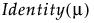Cauchy μ, σExponential μ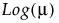Gamma μ, σBeta μ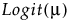Binomial n, p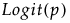Beta Binomial n, p, δPoisson λ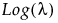Negative Binomial μ, σZero-Inflated Binomial n, p, π (zero-inflation)Zero-Inflated Beta Binomial n, p, δ, π (zero-inflation)Zero-Inflated Poisson λ, π (zero-inflation)Zero-Inflated Negative Binomial μ, σ, π (zero-inflation)Zero-Inflated Gamma μ, σ, π (zero-inflation)After selecting an appropriate Distribution, click Run. The Generalized Regression Model Launch panel opens.
 • Estimation Method Options
 • Advanced Controls
 • Validation Method Options
 • Early Stopping
 • Go
 • the l1 penalty, which penalizes the sum of the absolute values of the regression coefficients
 • the l2 penalty, which penalizes the sum of the squares of the regression coefficients
Computes parameter estimates by applying an l1 penalty. Due to the l1 penalty, some coefficients can be estimated as zero. Thus, variable selection is performed as part of the fitting procedure. In the ordinary Lasso, all coefficients are equally penalized. See Statistical Details for Estimation Methods.
Computes parameter estimates by penalizing a weighted sum of the absolute values of the regression coefficients. The weights in the l1 penalty are determined by the data in such as way as to guarantee the oracle property (Zou, 2006). This option uses the MLEs to weight the l1 penalty. MLEs cannot be computed when the number of predictors exceeds the number of observations or when there are strict linear dependencies among the predictors. If MLEs for the regression parameters cannot be computed, a generalized inverse solution or a ridge solution is used for the l1 penalty weights. See Statistical Details for Estimation Methods.
Computes parameter estimates by applying both an l1 penalty and an l2 penalty. The l1 penalty ensures that variable selection is performed. The l2 penalty improves predictive ability by shrinking the coefficients as ridge does. See Statistical Details for Estimation Methods.
Computes parameter estimates using an adaptive l1 penalty as well as an l2 penalty. This option uses the MLEs to weight the l1 penalty. MLEs cannot be computed when the number of predictors exceeds the number of observations or when there are strict linear dependencies among the predictors. If MLEs for the regression parameters cannot be computed, a generalized inverse solution or a ridge solution is used for the l1 penalty weights. You can set a value for the Elastic Net Alpha in the Advanced Controls panel. See Statistical Details for Estimation Methods.
 • When the tuning parameter is too small, the data are typically overfit and result in models with high variance.
 • When the tuning parameter is too large, the data are typically underfit.
 • For the Lasso, Elastic Net with Elastic Net Alpha specified, and Ridge, the value of the tuning parameter that gives the solution is the one that provides the best fit over the grid of tuning parameters.
 • If the design matrix is singular, the maximum likelihood estimates cannot be computed. The lower bound of 0.01 allows estimates close to the MLEs to be computed.
 • If the selected distribution is binomial, the lower bound of 0.01 helps prevent separation.
Requires lower-order effects to enter the model before their related higher order effects. In most cases, this means that X2 is not in the model unless X is in the model. For estimation methods other than Forward Selection, however, it is possible for X2 to enter the model and X to leave the model in the same step. If the data table contains a DOE script, this option is enabled, but it is off by default.
Sets the α parameter for the Elastic Net. This α parameter determines the mix of the l1 and l2  penalty tuning parameters in estimating the Elastic Net coefficients. The default value is α = 0.9, which sets the coefficient on the l1 penalty to 0.9 and the coefficient on the l2 penalty to 0.1. This option is available only when Elastic Net is selected as the Estimation Method. See Statistical Details for Estimation Methods.
 ‒ The observations are partitioned into k subsets, or folds.
 ‒ In turn, each fold is used as a validation set. A model is fit to the observations not in the fold. The LogLikelihood based on that model is calculated for the observations in the fold, providing a validation LogLikelihood.
 ‒ The mean of the validation LogLikelihoods for the k folds is calculated. This value serves as a validation LogLikelihood for the value of the tuning parameter.
The value of the tuning parameter that has the maximum validation LogLikelihood is used to construct the final solution. To obtain the final model, all k models derived for the optimal value of the tuning parameter are fit to the entire data set. Of these, the model that has the highest LogLikelihood is selected as the final model. The training set used for that final model is designated as the Training set and the holdout fold for that model is the Validation set. These are the Training and Validations sets used in plots and results in the report for the final solution.
When you click Go, a report opens. The title of the report specifies the fitting and validation methods that you selected. You can return to the Model Launch window to perform additional analyses and choose other estimation and validation methods.